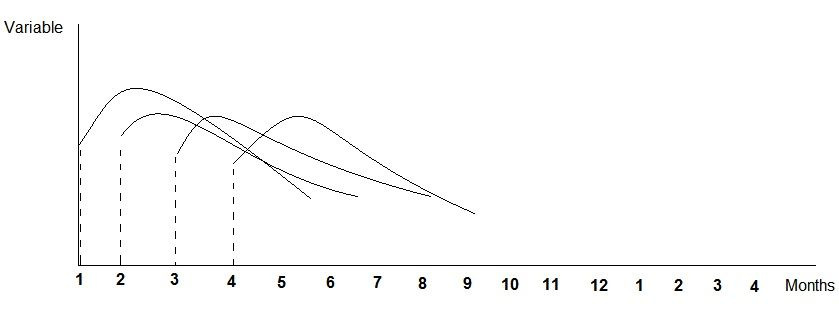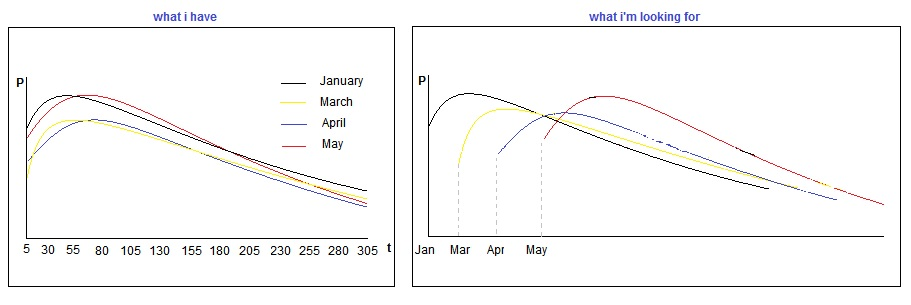## Different curve plotting

Hi,

I have a function of the form: P=a*(t**b)*exp(-c*t)
What I'm looking for is how to draw different curves depending on the month of the beginning of the action and have a tracing that looks like the attached model.
My data are as follows:

Id     a     b     c      month     P

a ranges from 3 to 50 and mean=13

b ranges from 0.01 to 1 and mean=0.27

c ranges from 0.0001 to 0.04 and mean=0.0045

t ranges from 1 to 305.

The curves to be plotted do not interest each id but id groups that have the same month of the beginning of the action.

My SAS version is 9.07 REPLIES 7

## Re: Different curve plotting

Did you really mean to say SAS 9.0?  Please confirm. I assume you have SAS/GRAPH?

If so, then according to the article "How old is your version of SAS," that version of SAS was shipped in 2002. There have been 11 releases of SAS in the last 14 years, with lots of awesome ways to solve this problem.

## Re: Different curve plotting

Yes, unfortunately, this is the only version we have in our lab. I downloaded SAS University Edition but I did not find myself comfortable with it.

## Re: Different curve plotting

I believe that your data description is incomplete. You say you have a formula P=a*(t**b)*exp(-c*t). You do not have a variable t mentioned in your data.

Also if "month" is supposed to be the month of something beginning (also not obvious) then you will need a different variable for your x axis, which appears to be dates.

Are you requesting help on how to take a set of parameters (a b and c) and generate a series of "t" values and then actually calculate ?

I would help to actually show some of your data.

## Re: Different curve plotting

Yes, you are absolutely right. For the tracing of the curve "t" is set to pitch values of 5 starting from 5 to 305.

Let me explain,
In fact the values of P are productions calculated from the parameters a b and c. P evolves according to time "t" and I can follow the tendency with a curve.
What I seek is to put on a single graph the productions of individuals who started their activity in January, those of February etc ... but different axes with steps of 1 month. Thank you for your interest in my question.

## Re: Different curve plotting

What UNIT is T measured in? what interval?

The do loop in this example is one basic way to generate the data for the curve for each set of parameters in your data.

```data example;
month=1;
a= 3;
b= 4;
c= 5;
do t=5 to 305;
P=a*(t**b)*exp(-c*t);
output;
end;
run; ```

To generate something that is meaningful in terms of "months" you are going to be involved with DATE manipulation some where, otherwise you won't get a transition for Month=12 to Month=1 as shown on your axis. We would need what the T is supposed to be, especially if it is not simpliy "days".

## Re: Different curve plotting

I did it  for each month of beginning of the execice (production) and in this case the abscissa axis is mentioned in day from 5 to 305, but the curves being on packed and the comparison is not easy. So I want to have the same curves (according to the starting months of the exercise) shifted by one step and each step represents the beginning month of the exercise as following:## Re: Different curve plotting

This may get you started

```data parm ;
input id a b c month;
datalines;
1  5  0.2 0.003 1
2  8  0.5 0.01  2
;
run;
data plot;
set parm;
plotstart= mdy(month,1,1960);
do t=5 to 305;
Plotdate= plotstart+t;
P=a*(t**b)*exp(-c*t);
if t=5 then Ny=p;
else NY=.;
output;
end;
/* to use the BREAK option in series plot so each
"months" series isn't connected to the other
we need a missing Y value*/
plotdate=plotdate+1;
p=.;
output;
run;
ods graphics on;
proc sgplot data=plot;
series x=plotdate y=p/break name='L' ;
needle x=plotdate y=ny/lineattrs=(pattern=4) name='N';
format Plotdate monname3.;
keylegend 'L';
run;
```

There are additional attribute data sets to set things like line colors associated with values of variables but you'll have to investigate those on your own.

Discussion stats
• 7 replies
• 789 views
• 0 likes
• 3 in conversation